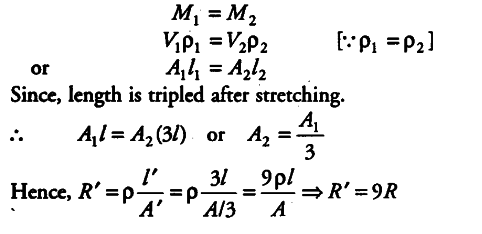# A conductor of length l

A conductor of length l is connected to a DC source of potential V. If the length of the conductor is tripled by gradually stretching it, keeping V constant, how will
(i) drift speed of electrons and
(ii) resistance of the conductor be affected?

The potential, V = constant, l’ = 3l
(i) Drift sped of electrons, v_{ d } = V/nelp
v_{ d } ∝ 1/l
so,when length is tripled,drift velocity gets one-third.
(ii) Resistance of conductor is R = p l/A
Here wire is stretched to trople its legth, that means the mass of the wire remains same in both conditions.
Before stretching mass = After stretchig massThus, new resistance is 9 times of its original value.